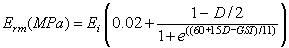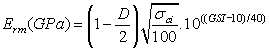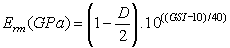Join one of our upcoming courses taking place around the world! Explore now

# Modulus Estimation Method

The deformation modulus (Young's Modulus) of a rock mass is often not a well known or easily measured parameter. Several researchers have proposed empirical relationships for estimating the rock mass deformation modulus on the basis of classification schemes.

In the RocSupport Project Settings dialog, you may select one of three methods for estimating the rock mass deformation modulus. These methods are based on the GSI rock mass classification scheme and the Generalized Hoek-Brown strength criterion. The applicable equations are described below.

The application of the Modulus Estimation Method in RocSupport depends on the Solution Method, Analysis Type and other selections in the input data dialogs.

Simplified Hoek & Diederichs

The Simplified Hoek & Diederichs equation for rock mass modulus, (Hoek & Diederichs (2006)), only requires GSI and D (Disturbance Factor) as input parameters. The modulus is calculated in MPa.Eqn.1

This equation is useful if reliable values of intact rock modulus are NOT available.

## Generalized Hoek & Diederichs

The detailed Hoek and Diederichs equation includes the modulus of the intact rock (Hoek & Diederichs (2006)), as well as GSI and D. The modulus is calculated in MPa.Eqn.2

This equation is recommended if reliable values of intact rock modulus are available.

## Hoek, Carranza-Torres, Corkum

The Hoek, Carranza-Torres and Corkum equation for rock mass modulus (Hoek, Carranza-Torres and Corkum (2002)), uses the uniaxial compressive strength of the intact rock (sigci), GSI and D.

For sigci <= 100 MPa, Equation 3 is used:Eqn.3

For sigci > 100 MPa, Equation 4 is used:Eqn.4

The modulus is calculated in GPa.